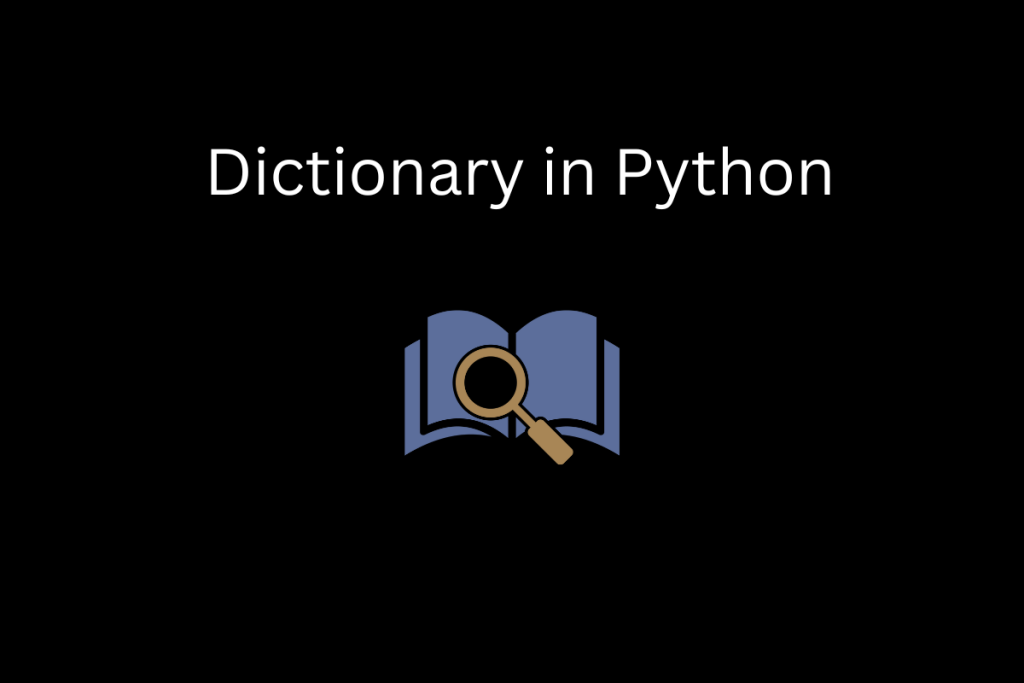# How to Initialize Dictionary in Python – A Step-By-Step GuideYou’re here because you want to learn how to initialize a dictionary in Python. And I’m here to show you how to do it.

## What is a dictionary in python ?

In Python, Dictionary is an unordered collection of items containing keys and values in pair. We can better understand by following an example.

```dictionary = { 1:"integer", 2.03:"Decimal", "Lion":"Animal"}
```

In the above dictionary:

• “integer” is a value of key “1”
• “Decimal” is a value of key “2.03”
• “Animal” is a value of key “Lion”

## Different ways to initialize a Python dictionary

We can define or initialize our dictionary using different methods in python as follows.

### Initializing Dictionary by passing literals

We can create a dictionary by passing key-value pairs as literals. The syntax for passing literals is given below following an appropriate example.

```dictionary_name = {
key1 : value1,
key2 : value2,
key3 : value3
}
```
```my_dictionary = {
"Lion" : "Animal",
"Parrot" : "Bird",
"Cobra" : "Reptile",
"Human" : "Mammals"
}
print(my_dictionary)
```

Our dictionary named “`my_dictionary`” will be created like this,

```{'Lion': 'Animal', 'Parrot': 'Bird', 'Cobra': 'Reptile', 'Human': 'Mammals'}
```

### Initializing Dictionary using the dict() constructor

We can create a dictionary using `dict`() constructor as follows.

```my_dictionary = dict(
Lion = "Animal",
Parrot = "Bird",
Cobra = "Reptile",
Human = 'Mammals'
)
print(my_dictionary)
```

Our dictionary will be created exactly like the previous method.

{‘Lion’: ‘Animal’, ‘Parrot’: ‘Bird’, ‘Cobra’: ‘Reptile’, ‘Human’: ‘Mammals’}

### Initializing dictionary using lists

We can create Dictionary by using two different lists of keys and values respectively as follows.

Let us create two different lists of keys and values respectively by following the code snippet.

```Keys = ["Lion", "Parrot", "Cobra", "Human"]
Values = ["Animal", "Bird", "Reptile", "Mammals"]
```

We are going to use `zip`() method inside `dict`() constructor as follows.

```my_dict = dict(zip(Keys,Values ))
print(my_dict)
```

Our dictionary will be created as follows.below

```{1: 'Integer', 2.0: 'Decimal', 'Lion': 'Animal', 'Parrot': 'Bird'}
```

Also read: How to convert a list to a dictionary in Python?

### Initializing Dictionary using Tuples

We can create a dictionary using tuples. A tuple is a collection of multiple items in a single variable irrespective of datatypes. It can store one or more values of different datatypes. We can create a Dictionary by using Tuples. Let us create a list of tuples first.

```Tuples = [(1 , "Integer"), (2.0 , "Decimal"), ("Lion" , "Animal"),("Parrot" , "Bird")]
My_dict = dict(Tuples)
print(My_dict)
```

In the above code snippet, `(1 , "Integer"), (2.0 , "Decimal"), ("Lion" , "Animal")` & `("Parrot" , "Bird")` are tuples.

We had created a list of tuples named “`Tuples`“. We created our required `Dictionary` by passing “`Tuple`” as parameter in `dict`() constructor. Our dictionary is created as follows.

```{1: 'Integer', 2.0: 'Decimal', 'Lion': 'Animal', 'Parrot': 'Bird'}
```

### Initializing dictionary using `__setitem__ `method

We can create a dictionary using `__setitem__ `method by following the code snippet given below. For the first, we need to create an empty dictionary.

```dict2 = {}
```

We need to input the keys as well as values into dict2 by using the `__setitem__` method as follows.

```dict2.__setitem__('key1', 'GEEK')
dict2.__setitem__('key2', 'God')
dict2.__setitem__('key3', 'is')
dict2.__setitem__('key4', 'Great')
print(dict2)
```

Our Dictionary will be created like this,

```{'key1': 'GEEK', 'key2': 'God', 'key3': 'is', 'key4': 'Great'}
```

### Initializing Dictionary using the `fromkeys`() method

We can use fromkeys() method to assign a common value to certain keys in a dictionary.

Let us take a list of keys and using `fromkeys`() method as follows:

```new_key = ["James", "Potter", "mathew"]
dict3 = dict.fromkeys(new_key, "men")
print(dict3)
```

The print function should print the dictionary for the above code snippet .

```{'James': 'men', 'Potter': 'men', 'mathew': 'men'}
```

instead of using `fromkeys`() method, we can also apply a for loop to get the same output as above.

```dict4={}
for x in new_key:
dict4[x] = "Men"
```

We can get the same dictionary.

```{'James': 'men', 'Potter': 'men', 'mathew': 'men'}
```

### Initializing Dictionary using `setdefault`() method

We can use setdefault() method to initialize dictionary in python as follows. This method returns the value of a key within a list comprehension.

Let us create an empty dictionary named `my_dictionary `and using `setdefault`() method to assign null as respective values for respective keys.

```my_dictionary = {}
[my_dictionary.setdefault(i, []) for i in range(4)]
```

Again, appending respective required values in our dictionary.

```my_dictionary.append('George')
my_dictionary.append('John')
print(my_dictionary)
```

We can get our dictionary as follows.

```{0: ['George'], 1: ['John'], 2: [], 3: []}
```

Again, appending one more value :

```my_dictionary.append('hrithik')
print(my_dictionary)
```

Again, We can get our dictionary as follows.

```{0: ['George'], 1: ['John'], 2: [], 3: ['hrithik'] }
```

### Initializing an empty dictionary

We can initialize an empty dictionary by following the code snippet.

```My_dict = {}
```

It will create an empty dictionary.

```print(My_dict)
{}
```